Home Practice
For learners and parents For teachers and schools
Textbooks
Full catalogue
Pricing SupportLog in

We think you are located in United States. Is this correct?

# Chapter 4: Intermolecular forces

If there were no intermolecular forces than all matter would exist as gases and we would not be here. This chapter introduces learners to a new concept called an intermolecular force. It is easy for learners to become confused as to whether they are talking about bonds or about intermolecular forces, particularly when the intermolecular forces in the noble gases are discussed. For this reason you should try and use the word bond or bonding to refer to the interatomic forces (the things holding the atoms together) and intermolecular forces for the things holding the molecules together. Getting learners to label the bonds and intermolecular forces on diagrams of molecules will help them to come to grips with the terminology.

This topic comes right after learners have learnt about electronegativity and polarity so this is a good chapter to reinforce those concepts and help learners see the use of electronegativity and polarity. Learners need to be very comfortable with determining the polarity and shape of molecules as this will help them determine the kinds of intermolecular forces that occur.

A brief overview of the topics covered in this chapter follows.

• What are intermolecular and how do they differ from bonds (interatomic forces).

This topic introduces learners to the concept of intermolecular forces. The five different types of intermolecular forces are introduced. Intermolecular forces are one of the main reason that matter exists in different states (solids, liquids and gases). Gases have no intermolecular forces between particles. For this reason you should either choose examples that are all in the liquid or solid state at room temperature (this temperature is the most familiar to learner) or remind learners that although the examples may be gases, we can consider the intermolecular forces between gases when they are cooled down and become liquids. It is also important to take care if you use the noble gases to explain induced dipole forces since technically these forces are not between molecules and so may confuse learners.

• Physical state and density.

Although this is listed as a separate point in CAPs, in this book it has been worked into the explanation of intermolecular forces. Solids have the strongest intermolecular forces between molecules and it is these forces which hold the molecules in a rigid shape. In a liquid the intermolecular forces are continuously breaking and reforming as the molecules move and slide over each other.

• Particle kinetic energy and temperature.

This topic is also listed as a separate point in CAPs and is worked into the explanation of intermolecular forces. This topic links back to grade $$\text{10}$$ (states of matter and the kinetic molecular theory) and also links to chapter $$\text{7}$$ (ideal gases).

• The chemistry of water.

The second half of this chapter is devoted to understanding more about water. Water is a unique liquid in many aspects. Some of these properties of water are explained in this part of the text. It is important to link this into intermolecular forces and in particular the strong hydrogen bonds that are found between water molecules.

All around us we see matter in different phases. The air we breathe is a gas, while the water you drink is a liquid and the chair you are sitting on is a solid. In this chapter we are going to look at one of the reasons that matter exists as solids and liquids.

In the previous chapter, we discussed the different forces that exist between atoms (interatomic forces). When atoms are joined to one another they form molecules, and these molecules in turn have forces that bind them together. These forces are known as intermolecular forces.

Intermolecular forces allow us to determine which substances are likely to dissolve in which other substances and what the melting and boiling points of substances are. Without intermolecular forces holding molecules together we would not exist.

Note that we will use the term molecule throughout this chapter as the compounds we are looking at are all covalently bonded and do not exist as giant networks (recall from grade $$\text{10}$$ that there are three types of bonding: metallic, ionic and covalent). Sometimes you will see the term simple molecule. This is a covalent molecular structure.

Interatomic (between atoms) forces are also known as intramolecular (within molecules) forces. You can remember this by thinking of international which means between nations.

## 4.1 Intermolecular and interatomic forces (ESBMM)

Intermolecular forces

Intermolecular forces are forces that act between molecules.

You will also recall from the previous chapter, that we can describe molecules as being either polar or non-polar. A polar molecule is one in which there is a difference in electronegativity between the atoms in the molecule, such that the shared electron pair spends more time close to the atom that attracts it more strongly. The result is that one end of the molecule will have a slightly positive charge ($$δ^{+}$$), and the other end will have a slightly negative charge ($$δ^{-}$$). The molecule is said to be a dipole.

A dipole molecule is a molecule that has two (di) poles. One end of the molecule is slightly positive and the other is slightly negative. We can depict this very simply as an oval with one positive side and one negative. In reality however, the molecules do not look like this, they look more like the images in Figure 4.1.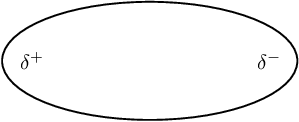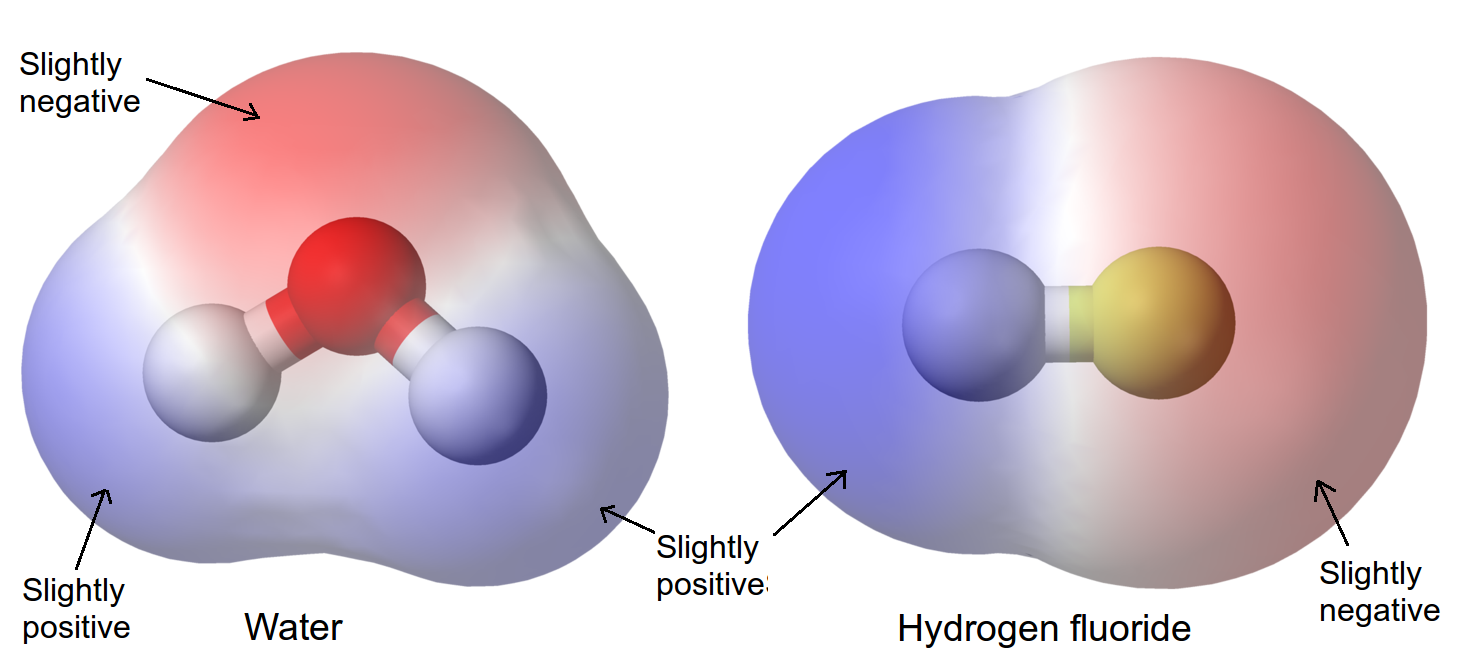Figure 4.1: A different representation of dipole molecules. The red region is slightly negative, and the blue region is slightly positive.

It is important to remember that just because the bonds within a molecule are polar, the molecule itself may not necessarily be polar. The shape of the molecule may also affect its polarity. A few examples are shown in Table 4.1 to refresh your memory. Note that we have shown tetrahedral molecules with all the terminal atoms at $$\text{90}$$$$\text{°}$$ to each other (i.e. flat or 2-dimensional), but the shape is really 3-dimensional.

 Molecule Chemical formula Bond between atoms Shape of molecule Polarity of molecule Hydrogen $$\text{H}_{2}$$ Non-polar covalent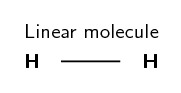Non-polar Hydrogen chloride $$\text{HCl}$$ Polar covalent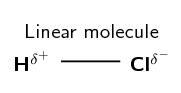Polar Carbon tetrafluoride $$\text{CF}_{4}$$ Polar covalent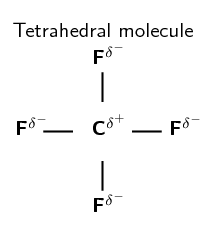Non-polar Trifluoro-methane $$\text{CHF}_{3}$$ Polar covalent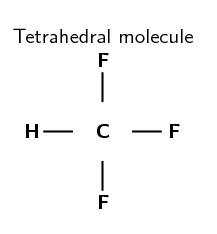Polar
Table 4.1: Polarity in molecules with different atomic bonds and molecular shapes.

### Types of intermolecular forces (ESBMN)

It is important to be able to recognise whether the molecules in a substance are polar or non-polar because this will determine what type of intermolecular forces there are. This is important in explaining the properties of the substance.

1. Ion-dipole forces

As the name suggests, this type of intermolecular force exists between an ion and a dipole (polar) molecule. You will remember that an ion is a charged atom, and this will be attracted to one of the charged ends of the polar molecule. A positive ion will be attracted to the negative pole of the polar molecule, while a negative ion will be attracted to the positive pole of the polar molecule. This can be seen when sodium chloride ($$\text{NaCl}$$) dissolves in water. The positive sodium ion ($$\text{Na}^{+}$$) will be attracted to the slightly negative oxygen atoms in the water molecule, while the negative chloride ion ($$\text{Cl}^{-}$$) is attracted to the slightly positive hydrogen atoms. These intermolecular forces weaken the ionic bonds between the sodium and chloride ions so that the sodium chloride dissolves in the water (Figure 4.2).

This is a simplified diagram to highlight the regions of positive and negative charge. When sodium chloride dissolves in water it can more accurately be shown as:

2. Ion-induced-dipole forces

Similar to ion-dipole forces these forces exist between ions and non-polar molecules. The ion induces a dipole in the non-polar molecule leading to a weak, short lived force which holds the compounds together.

These forces are found in haemoglobin (the molecule that carries oxygen around your body). Haemoglobin has $$\text{Fe}^{2+}$$ ions. Oxygen ($$\text{O}_{2}$$) is attracted to these ions by ion-induced dipole forces.

3. Dipole-dipole forces

When one dipole molecule comes into contact with another dipole molecule, the positive pole of the one molecule will be attracted to the negative pole of the other, and the molecules will be held together in this way (Figure 4.3). Examples of materials/substances that are held together by dipole-dipole forces are $$\text{HCl}$$, $$\text{SO}_{2}$$ and $$\text{CH}_{3}\text{Cl}$$.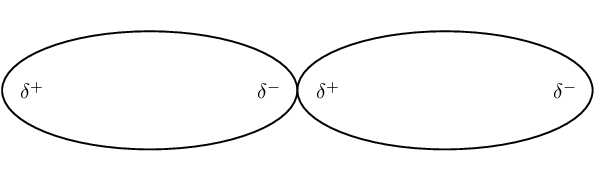Figure 4.3: Two dipole molecules are held together by the attractive force between their oppositely charged poles.

One special case of this is hydrogen bonding.

4. Induced dipole forces

These intermolecular forces are also sometimes called “London forces” or “momentary dipole” forces or “dispersion” forces.

We know that while carbon dioxide is a non-polar molecule, we can still freeze it (and we can also freeze all other non-polar substances). This tells us that there must be some kind of attractive force in these kinds of molecules (molecules can only be solids or liquids if there are attractive forces pulling them together). This force is known as an induced dipole force.

In non-polar molecules the electronic charge is usually evenly distributed but it is possible that at a particular moment in time, the electrons might not be evenly distributed (remember that the electrons are always moving in their orbitals). The molecule will have a temporary dipole. In other words, each end of the molecules has a slight charge, either positive or negative. When this happens, molecules that are next to each other attract each other very weakly. These forces are found in the halogens (e.g. $$\text{F}_{2}$$ and $$\text{I}_{2}$$) and in other non-polar molecules such as carbon dioxide and carbon tetrachloride.

All covalent molecules have induced dipole forces. For non-polar covalent molecules these forces are the only intermolecular forces. For polar covalent molecules, dipole-dipole forces are found in addition to the induced dipole forces.

When the noble gases condense, the intermolecular forces that hold the liquid together are induced dipole forces.

5. Dipole-induced-dipole forces

This type of force occurs when a molecule with a dipole induces a dipole in a non-polar molecule. It is similar to an ion-induced dipole force. An example of this type of force is chloroform ($$\text{CHCl}_{3}$$) in carbon tetrachloride ($$\text{CCl}_{4}$$).

The following image shows the types of intermolecular forces and the kinds of compounds that lead to those forces.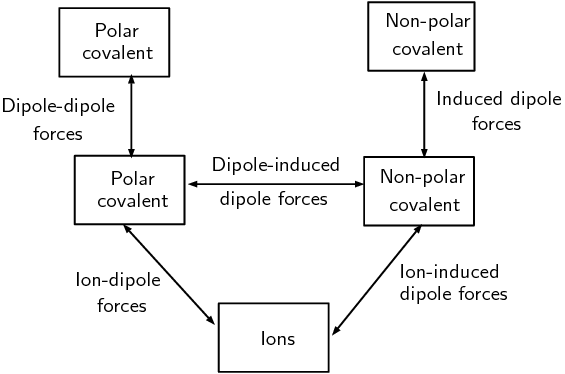Figure 4.4: The types of intermolecular forces. The boxes represent the type of compound while the lines represent the type of force.

The last three forces (dipole-dipole forces, dipole-induced dipole forces and induced dipole forces) are sometimes collectively known as van der Waals' forces. We will now look at a special case of dipole-dipole forces in more detail.

Hydrogen bonds

As the name implies, this type of intermolecular bond involves a hydrogen atom. When a molecule contains a hydrogen atom covalently bonded to a highly electronegative atom ($$\text{O}$$, $$\text{N}$$ or $$\text{F}$$) this type of intermolecular force can occur. The highly electronegative atom on one molecule attracts the hydrogen atom on a nearby molecule.

Water molecules for example, are held together by hydrogen bonds between the hydrogen atom of one molecule and the oxygen atom of another (Figure 4.5). Hydrogen bonds are a relatively strong intermolecular force and are stronger than other dipole-dipole forces. It is important to note however, that hydrogen bonds are weaker than the covalent and ionic bonds that exist between atoms.

Do not confuse hydrogen bonds with actual chemical bonds. Hydrogen bonding is an example of a case where a scientist named something believing it to be one thing when in fact it was another. In this case the strength of the hydrogen bonds misled scientists into thinking this was actually a chemical bond, when it is really just an intermolecular force.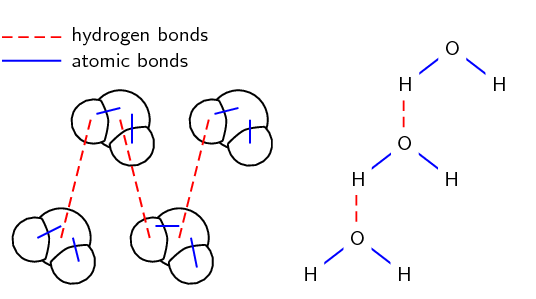Figure 4.5: Two representations showing the hydrogen bonds between water molecules: space-filling model and structural formula.

### The difference between intermolecular and interatomic forces (ESBMP)

It is important to realise that there is a difference between the types of interactions that occur in molecules and the types that occur between molecules. In the previous chapter we focused on the interactions between atoms. These are known as interatomic forces or chemical bonds. We also studied covalent molecules in more detail.

Remember that a covalent bond has an electronegativity difference of less than $$\text{2,1}$$. Covalent molecules have covalent bonds between their atoms. Van der Waals' forces only occur in covalent molecules. We can show the interatomic and intermolecular forces between covalent compounds diagrammatically or in words. Intermolecular forces occur between molecules and do not involve individual atoms. Interatomic forces are the forces that hold the the atoms in molecules together. Figure 4.5 shows this.

 Interatomic forces Intermolecular forces Atoms or molecules Forces between atoms Forces between molecules Strength of forces Strong forces Relatively weak forces Distance between atoms or molecules Very short distances Larger distances than bonds
Table 4.2: The differences between interatomic and intermolecular forces.

The worked examples on intermolecular forces condense a lot of information into the first step. You may need to remind learners how to determine molecular polarity. To do this, you can use the worked examples in atomic combinations as a quick refresher of the topic. In tests and exams learners need to be able to quickly identify a polar or non-polar molecule and so need to be very comfortable with this skill.

## Worked example 1: Intermolecular forces

Which intermolecular forces are found in carbon tetrachloride ($$\text{CCl}_{4}$$)?

Carbon has an electronegativity of $$\text{2,5}$$. Chlorine has an electronegativity of $$\text{3,0}$$. The electronegativity difference between carbon and chlorine is $$\text{1,0}$$ (recall the section on electronegativity in the previous chapter). We also know that the bond between carbon and chlorine is polar.

Also from the previous chapter we know that carbon tetrachloride is a tetrahedral molecule (recall molecular shape). Carbon tetrachloride is symmetrical and so is non-polar overall.

### Now decide which case it is

Carbon tetrachloride is non-polar and so the only kind of force that can exist is induced dipole.

## Worked example 2: Intermolecular forces

Which intermolecular forces are found in the following solution: sodium chloride in water?

Sodium chloride is ionic. (the electronegativity difference is $$\text{2,1}$$). Water has polar bonds (the electronegativity difference is $$\text{1,4}$$). Water is a polar molecule (its molecular shape is bent or angular).

### Now decide which case it is

This is an ionic substance interacting with a polar substance. This interaction is an ion-dipole force.

Textbook Exercise 4.1

Which intermolecular forces are found in:

hydrogen fluoride ($$\text{HF}$$)

Hydrogen fluoride is a polar covalent molecule. (It is linear and not symmetrical.) So the type of intermolecular force is dipole-dipole forces.

methane ($$\text{CH}_{4}$$)

Methane is a non-polar covalent molecule. (It is tetrahedral and symmetrical.) So the type of intermolecular force is induced dipole forces.

potassium chloride in ammonia ($$\text{KCl}$$ in $$\text{NH}_{3}$$)

Potassium chloride is an ionic compound. Ammonia is a polar covalent molecule. (It is trigonal pyramidal and not symmetrical.) So the type of intermolecular force is ion-dipole forces.

krypton ($$\text{Kr}$$)

Krypton is a noble gas. So the type of intermolecular force is induced dipole forces.

### Understanding intermolecular forces (ESBMQ)

The types of intermolecular forces that occur in a substance will affect its properties, such as its phase, melting point and boiling point. You should remember from the kinetic theory of matter (see grade $$\text{10}$$), that the phase of a substance is determined by how strong the forces are between its particles. The weaker the forces, the more likely the substance is to exist as a gas. This is because the particles are able to move far apart since they are not held together very strongly. If the forces are very strong, the particles are held closely together in a solid structure. Remember also that the temperature of a material affects the energy of its particles. The more energy the particles have, the more likely they are to be able to overcome the forces that are holding them together. This can cause a change in phase.

Shown below are the three phases of water. Note that we are showing two dimensional figures when in reality these are three dimensional.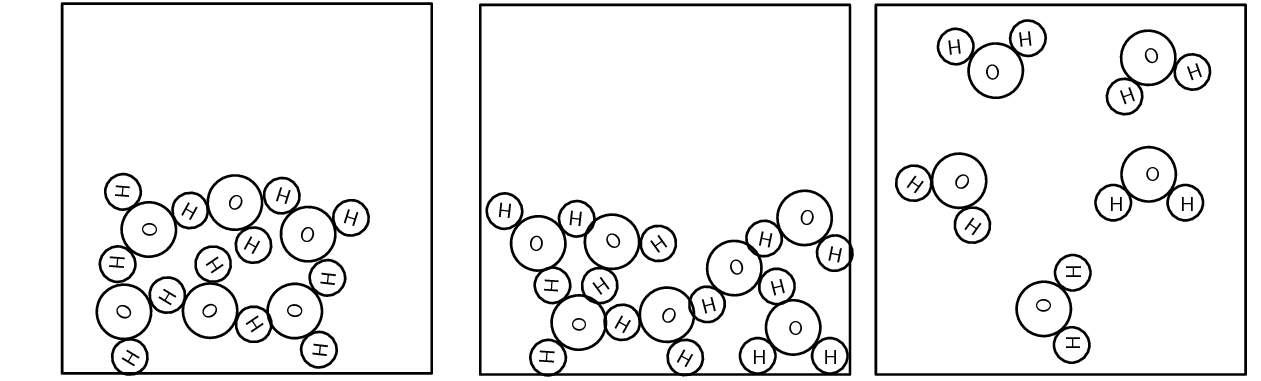Figure 4.6: The three phases of water.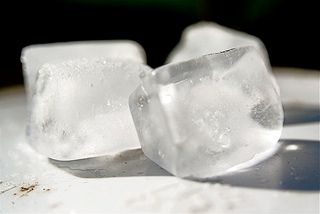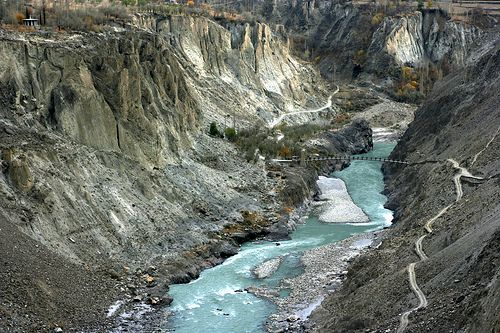#### The effects of intermolecular forces

The following five experiments investigate the effect of various physical properties (evaporation, surface tension, solubility, boiling point and capillarity) of substances and determine how these properties relate to intermolecular forces. Each experiment will look at a different property.

A formal experiment on the effects of intermolecular forces is included in this chapter. In this experiment learners will investigate how intermolecular forces affect evaporation, surface tension, solubility, boiling points and capillarity. Some of the substances that are used (nail polish remover (mainly acetone if you use the non acetone free variety), methylated spirits (a mixture of methanol and ethanol), oil (a mostly non-polar hydrocarbon), glycerin (a fairly complex organic molecule)) are quite complex substances and learners may not have the skills needed to determine the types of intermolecular forces at work here. You should guide learners in this and tell them the intermolecular forces for these substances.

You can help learners work out the strength of the intermolecular forces by telling them that larger molecules have stronger intermolecular forces than smaller molecules. This is often a big factor in determining which substance has the strongest intermolecular forces.

This experiment is split into five experiments. Each experiment focuses on a different property and sees how that property relates to intermolecular forces. It will often not be easy for learners to see the small differences between some of the molecules chosen and so they need to use a combination of experimental results and knowledge about the strength of the intermolecular force to try and predict what may happen. Each experiment ends with a conclusion about what should be found to guide learners.

It is very important to work in a well ventilated room (one with lots of air flow) particularly when working with methanol and ethanol. Many of the substances used (particularly nail polish remover, ethanol and methylated spirits) are highly flammable and so care must be taken when heating these substances. It is recommended that learners use a hot plate rather than a Bunsen burner to heat these substances as this reduces the risk of fire. When doing chemistry experiments it is also extra important to ensure that your learners do not run around, do not try to drink the chemicals, do not eat and drink in the lab, do not throw chemicals on the other learners and in general do act in a responsible and safe way. The guidelines for safe experimental work can be found in the science skills chapter from grade $$\text{10}$$.

## The effects of intermolecular forces: Part $$\text{1}$$

### Aim

To investigate evaporation and to determine the relation between evaporation and intermolecular forces.

### Apparatus

You will need the following items for this experiment:

• ethanol, water, nail polish remover (acetone), methylated spirits

• evaporating dishes (or shallow basins)

### Method

1. Place $$\text{20}$$ $$\text{ml}$$ of each substance given in separate evaporating dishes.
2. Carefully move each dish to a warm (sunny) spot.
3. Mark the level of liquid in each dish using a permanent marker. Make several marks at different positions around the dish. If the permanent marker is leaving a smudge rather than a noticeable mark, carefully wipe the side of the dish and try again.
4. Observe each dish every minute and note which liquid evaporates fastest.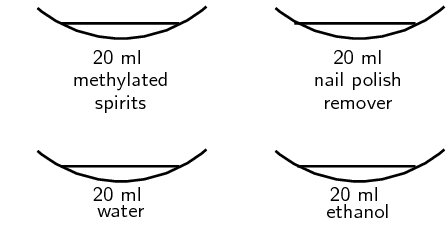### Results

Record your results in the table below. You do not need to measure the level of the liquid, but rather just write how much the level had dropped (e.g. for water you might write did not notice any decrease in the level or for ethanol you might write almost all the liquid had evaporated).

 Substance Liquid level after $$\text{1}$$ $$\text{min}$$ $$\text{2}$$ $$\text{min}$$ $$\text{3}$$ $$\text{min}$$ $$\text{4}$$ $$\text{min}$$ $$\text{5}$$ $$\text{min}$$ Ethanol Water Nail polish remover Methylated spirits

### Discussion and conclusion

You should find that water takes the longest time to evaporate. Water has strong intermolecular forces (hydrogen bonds). Ethanol ($$\text{CH}_{3}\text{CH}_{2}\text{OH}$$) and methylated spirits (mainly ethanol ($$\text{CH}_{3}\text{CH}_{2}\text{OH}$$) with some methanol ($$\text{CH}_{3}\text{OH}$$)) both have hydrogen bonds but these are slightly weaker than the hydrogen bonds in water. Nail polish remover (acetone ($$\text{CH}_{3}\text{COCH}_{3}$$)) has dipole-dipole forces only and so evaporates quickly.

Substances with weaker intermolecular forces evaporate faster than substances with stronger intermolecular forces.

## The effects of intermolecular forces: Part $$\text{2}$$

### Aim

To investigate surface tension and to determine the relation between surface tension and intermolecular forces.

### Apparatus

You will need the following items for this experiment:

• water, cooking oil (sunflower oil), glycerin, nail polish remover (acetone), methylated spirits

• small glass beakers or glass measuring cylinders

• small piece of glass or clear plastic (about $$\text{5}$$ $$\text{cm}$$ by $$\text{5}$$ $$\text{cm}$$.)

### Method

1. Place about $$\text{50}$$ $$\text{ml}$$ of each substance given in separate small beakers or measuring cylinders.
2. Observe the shape of the meniscus. (This is the level of the liquid). Note what happens at the edges where the liquid touches the glass. (You can place a few drops of food colouring in each substance to help you see the meniscus.)
3. Now place a drop of the substance on a small piece of glass. Observe the shape of the drop.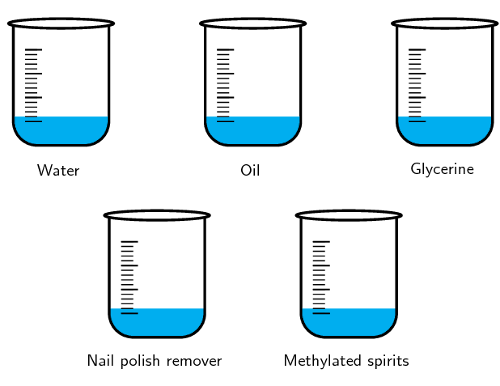### Results

Record your results in the table below. You just need to give a qualitative result (in other words what you see in the experiment).

 Substance Shape of meniscus Shape of droplet Water Oil Glycerine Nail polish remover Methylated spirits

### Discussion and conclusion

The meniscus for all these substances should be concave (i.e. higher at the edges than in the middle). This is because the forces holding the molecules in the substance together are weaker than the attraction between the substance and the glass of the tube.

You should also have noticed that water, oil and Glycerine tend to form a drop, while nail polish remover and methylated spirits do not. Strong intermolecular forces help hold the substance together, while weaker ones do not hold the molecules in the substance together as much.

Water has the strongest intermolecular forces (hydrogen bonds) of all the substances used. Glycerine and methylated spirits also have hydrogen bonds, but these intermolecular forces are slightly weaker than in water. Sunflower oil is mostly non-polar but has very long molecules which help account for the higher surface tension.

Substances with strong intermolecular forces will generally have a greater surface tension than substances with weaker intermolecular forces.

## The effects of intermolecular forces: Part $$\text{3}$$

### Aim

To investigate solubility and to determine the relation between solubility and intermolecular forces.

### Apparatus

You will need the following items for this experiment:

• Solids: sodium chloride (table salt), iodine, potassium permanganate

• Solvents: water, ethanol, chloroform
• $$\text{9}$$ beakers or test-tubes

• $$\text{3}$$ A4 sheets of paper

### Method

1. Place about $$\text{20}$$ $$\text{ml}$$ of each solvent given in separate beakers. Place this set on a piece of paper labelled “sodium chloride”.
2. Repeat this step twice. The second set is for potassium permanganate (so your piece of paper will say “potassium permanganate”) and the third set is for iodine (so your piece of paper will say “iodine”). You should now have nine beakers in total.
3. Into the first set, add about $$\text{2}$$ $$\text{g}$$ of sodium chloride.
4. Into the second set, add about $$\text{2}$$ $$\text{g}$$ of potassium permanganate.
5. Into the third set, add about $$\text{2}$$ $$\text{g}$$ of iodine.
6. Observe how much of each substance dissolves in the solvent.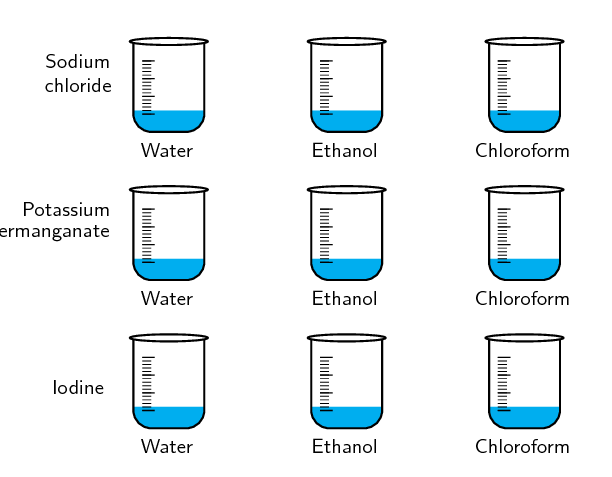### Results

Record your results in the table below. If you observe only a small amount of the solid dissolving then write that very little solid dissolved. If all the solid dissolves then write that all the solid dissolved.

 Substance Water Chloroform Ethanol Sodium chloride Potassium permanganate Iodine

### Discussion and conclusion

You should find that the sodium chloride and potassium permanganate dissolved (at least a bit) in all the substances. The iodine did not dissolve in any of the substances. The three solvents (water, chloroform and ethanol) are all polar and have dipole-dipole forces. Sodium chloride and potassium permanganate are both ionic substances, while iodine is non-polar.

Substances will dissolve in solvents that have similar intermolecular forces or in solvents where the ionic bonds can be disrupted by the formation of ion-dipole forces.

## The effects of intermolecular forces: Part $$\text{4}$$

### Aim

To investigate boiling point and to determine the relation between boiling point and intermolecular forces.

### Apparatus

You will need the following items for this experiment:

• water, cooking oil (sunflower oil), Glycerine, nail polish remover, methylated spirits

• test-tubes and a beaker

• hot plate

### Method

Methylated spirits and nail polish remover are highly flammable. They will easily catch fire if left near an open flame. For this reason they must be heated in a water bath. This experiment MUST be performed in a well ventilated room.

1. Place about $$\text{20}$$ $$\text{ml}$$ of each substance given in separate test-tubes.
2. Half-fill the beaker with water and place on the hot plate.
3. Place the test-tubes in the beaker.
4. Observe how long each substance takes to boil. As soon as a substance boils, remove it from the water bath.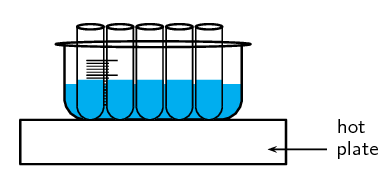### Results

Write down the order in which the substances boiled, starting with the substance that boiled first and ending with the substance that boiled last.

### Discussion and conclusion

You should have found that the nail polish remover and the methylated spirits boil before the water, oil and Glycerine.

Glycerine, water and methylated spirits have hydrogen bonds between the molecules. However, in water and Glycerine these intermolecular forces are very strong while in the methylated spirits they are slightly weaker. This leads to the higher boiling point for water and Glycerine. Nail polish remover has weaker dipole-dipole forces.

Although cooking oil is non-polar and has induced dipole forces the molecules are very large and so these increase the strength of the intermolecular forces.

Substances with strong intermolecular forces will have a higher boiling point than substances with weaker intermolecular forces.

## The effects of intermolecular forces: Part $$\text{5}$$

### Aim

To investigate capillarity (how far up a tube a liquid rises or how far down a liquid falls) and to determine the relation between capillarity and intermolecular forces.

### Apparatus

You will need the following items for this experiment:

• water, cooking oil (sunflower oil), nail polish remover, methylated spirits

• large shallow dish, narrow glass tube (with one end closed)

### Method

1. Place about $$\text{20}$$ $$\text{ml}$$ of water in the shallow dish.
2. Hold the narrow tube just above the level of the water in the dish.
3. Observe how far up the tube the water travels.
4. Repeat for the other three substances, remembering to wash and dry the dish and tube well between each one.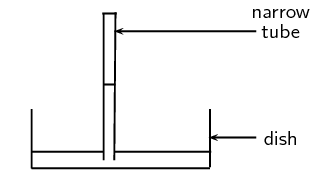### Results

Record your results in the table below. You do not need to measure how far up the tube the substance travels but rather say if it only travelled a short distance or a long distance.

 Substance Distance travelled up tube Water Oil Nail polish remover Methylated spirits

### Discussion and conclusion

Water travels the greatest distance up the tube. Nail polish remover travels the least distance.

Capillarity is related to surface tension. If the attractive force between the glass walls of the tube and the substance are stronger than the intermolecular forces in the substance, than the edges of the liquid will be pulled above the surface of the liquid. This in turn helps pull the liquid up the tube.

Substances with strong intermolecular forces will travel further up a narrow tube (have a greater capillarity) than substances with weaker intermolecular forces.

From these experiments we can see how intermolecular forces (a microscopic property) affect the macroscopic behaviour of substances. If a substance has weak intermolecular forces then it will evaporate easily. Substances with weak intermolecular forces also have low surface tension and do not rise as far up in narrow tubes as substances with strong intermolecular forces. Boiling points are lower for substances with weak intermolecular forces. Substances are more likely to be soluble in liquids with similar intermolecular forces.

We will now look at some more properties (molecular size, viscosity, density, melting and boiling points, thermal expansion, thermal conductivity) in detail.

Molecular size

The alkanes are a group of organic compounds that contain carbon and hydrogen bonded together. The carbon atoms link together to form chains of varying lengths.

The boiling point and melting point of these molecules is determined by their molecular structure, and their surface area. The more carbon atoms there are in an alkane, the greater the surface area and therefore the higher the boiling point. The melting point also increases as the number of carbon atoms in the molecule increases. This can be seen in the table below.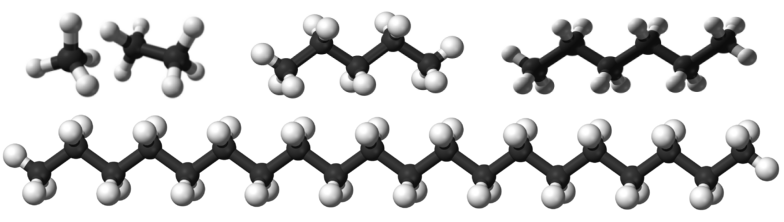Formula $$\text{CH}_{4}$$ $$\text{C}_{2}\text{H}_{6}$$ $$\text{C}_{5}\text{H}_{12}$$ $$\text{C}_{6}\text{H}_{14}$$ $$\text{C}_{20}\text{H}_{42}$$ Name methane ethane pentane hexane icosane Molecular mass ($$\text{g·mol^{-1}}$$) $$\text{16}$$ $$\text{30}$$ $$\text{72}$$ $$\text{86}$$ $$\text{282}$$ Melting point (℃) $$-\text{183}$$ $$-\text{183}$$ $$-\text{130}$$ $$-\text{95}$$ $$\text{37}$$ Boiling point (℃) $$-\text{164}$$ $$-\text{89}$$ $$\text{36}$$ $$\text{69}$$ $$\text{343}$$ Phase at room temperature gas gas liquid liquid solid

You will also notice that, when the molecular mass of the alkanes is low (i.e. there are few carbon atoms), the organic compounds are gases because the intermolecular forces are weak. As the number of carbon atoms and the molecular mass increases, the compounds are more likely to be liquids or solids because the intermolecular forces are stronger.

You should see that the larger a molecule is the stronger the intermolecular forces are between its molecules. This is one of the reasons why methane ($$\text{CH}_{4}$$) is a gas at room temperature while pentane ($$\text{C}_{5}\text{H}_{12}$$) is a liquid and icosane ($$\text{C}_{20}\text{H}_{42}$$) is a solid.

It is partly the stronger intermolecular forces that explain why petrol (mainly octane ($$\text{C}_{8}\text{H}_{18}$$)) is a liquid, while candle wax ($$\text{C}_{23}\text{H}_{48}$$) is a solid. If these intermolecular forces did not increase with increasing molecular size we would not be able to put liquid fuel into our cars or use solid candles.

Viscosity

Viscosity is the resistance to flow of a liquid. Compare how easy it is to pour water and syrup or honey. The water flows much faster than the syrup or honey.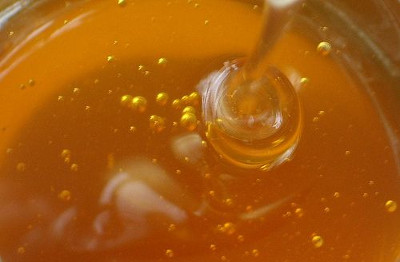You can see this if you take a cylinder filled with water and a cylinder filled with glycerin. Drop a small metal ball into each cylinder and note how easy it is for the ball to fall to the bottom. In the glycerin the ball falls slowly, while in the water it falls faster.

Substances with stronger intermolecular forces are more viscous than substances with weaker intermolecular forces.

## Machine and motor oils

You are given the following information about engine oils.

 Oil Use Other info SAE 30 monograde Engines Low viscosity SAE 50 monograde Engines High viscosity SAE 15W-40 multigrade Engines Medium viscosity SAE 0W-40 multigrade Engines Medium viscosity

(Data from shell.com)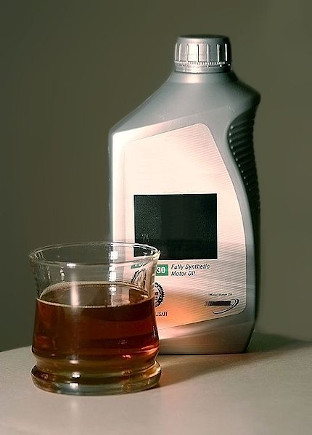Multigrade oils can be used even in cold weather since they remain fluid (The first number is the rating for winter weather and the W shows that this is the rating in winter. The second number is the viscosity rating in summer). Monograde oils are given their viscosity rating at $$\text{100}$$$$\text{°C}$$. The viscosity is an indication of how well the oil flows. The more viscous an oil the larger the molecules that are in the oil.

• Which oil has the longest molecules?

• Which oil has the shortest molecules?

• Which oil has the strongest overall intermolecular forces?

• Which oil has the weakest overall intermolecular forces?

• What can you conclude about the link between the magnitude of the intermolecular force and viscosity?

Density

Density
Density is a measure of the mass in a unit volume.

The solid phase is often the most dense phase (water is one noteworthy exception to this). This can be explained by the strong intermolecular forces found in a solid. These forces pull the molecules together which results in more molecules in one unit volume than in the liquid or gas phases. The more molecules in a unit volume the denser that substance will be.

Melting and boiling points

Intermolecular forces affect the boiling and melting points of substances. Substances with weak intermolecular forces will have low melting and boiling points while those with strong intermolecular forces will have high melting and boiling points. In the experiment on intermolecular forces you investigated the boiling points of several substances, and should have seen that molecules with weaker intermolecular forces have a lower boiling point than molecules with stronger intermolecular forces.

One further point to note is that covalent network structures (recall from grade $$\text{10}$$ that these are covalent compounds that form large networks and an example is diamond) will have high melting and boiling points due to the fact that some bonds (i.e. the strong forces between atoms) have to break before the substance can melt. Covalent molecular substances (e.g. water, sugar) often have lower melting and boiling points, because of the presence of the weaker intermolecular forces holding these molecules together.

Thermal expansion

As substances are heated their molecules start moving more vigorously (their kinetic energy increases). This causes the liquid to expand on heating. You can observe this in a thermometer. As the alcohol (or mercury) is heated it expands and rises up the tube.

This is why when you tile a floor you have to leave gaps between the tiles to allow for expansion. It is also why power lines sag slightly and bridges have slight gaps for expansion.

Thermal conductivity

Different materials conduct heat differently. The following activity will highlight this.

## Thermal conductivity

Take a long thin piece of graphite and a long thin piece of copper (or other metal). Attach a bit of wax to the one end of each rod (you will need to melt the wax a bit first to make it stick). While the wax is still soft, press a toothpick into the blob of wax.

Now suspend the graphite and copper rods from a desk or chair using a piece of string and heat the other end. Observe which toothpick falls off first. Try to explain why.

Heat is transferred through a substance from the point being heated to the other end. This is why the bottom of a pot gets hot first (assuming you are heating the pot on a stove plate). In metals there are some free, delocalised electrons which can help transfer the heat energy through the metal. In covalent molecular compounds there are no free, delocalised electrons and the heat does not travel as easily through the material.

## Worked example 3: Understanding intermolecular forces

Explain why the melting point of oxygen ($$\text{O}_{2}$$) is much lower than the melting point of hydrogen chloride $$\text{HCl}$$.

### Write down what you know about melting points and forces

The stronger the intermolecular force, the higher the melting point. So if a substance has strong intermolecular forces, then that substance will have a high melting point.

### Write down which forces occur in the two given compounds

Oxygen is non-polar and has induced dipole forces. Hydrogen chloride is polar and has dipole-dipole forces.

### Combine all the facts to get the answer

We know that stronger intermolecular forces lead to higher melting points. We also know that oxygen has weaker intermolecular forces than hydrogen chloride (induced dipole versus dipole-dipole forces). Therefore oxygen will have a lower melting point than hydrogen chloride since oxygen has weaker intermolecular forces.

## Types of intermolecular forces

Textbook Exercise 4.2

Given the following diagram: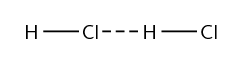1. Name the molecule and circle it on the diagram
2. Label the interatomic forces (covalent bonds)
3. Label the intermolecular forces

The molecule is hydrogen chloride.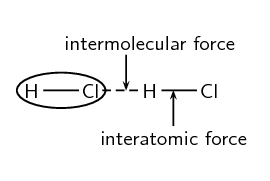Given the following molecules and solutions:

$$\text{HCl}$$, $$\text{CO}_{2}$$, $$\text{I}_{2}$$, $$\text{H}_{2}\text{O}$$, $$\text{KI} (\text{aq})$$, $$\text{NH}_{3}$$, $$\text{NaCl}(\text{aq})$$, $$\text{HF}$$, $$\text{MgCl}_{2}$$ in $$\text{CCl}_{4}$$, $$\text{NO}$$, $$\text{Ar}$$, $$\text{SiO}_{2}$$

Complete the table below by placing each molecule next to the correct type of intermolecular force.

 Ion-dipole Ion-induced-dipole Dipole-dipole (no hydrogen bonding) Dipole-dipole (hydrogen bonding) Induced dipole Dipole-induced-dipole

In which one of the substances listed above are the intermolecular forces:

1. strongest

2. weakest

 Ion-dipole $$\text{KI} (\text{aq})$$, $$\text{NaCl}(\text{aq})$$, $$\text{HF}(\text{aq})$$ Ion-induced-dipole $$\text{MgCl}_{2}$$ in $$\text{CCl}_{4}$$ Dipole-dipole (no hydrogen bonding) $$\text{HCl}$$, $$\text{NO}$$ Dipole-dipole (hydrogen bonding) $$\text{H}_{2}\text{O}$$, $$\text{NH}_{3}$$ Induced dipole $$\text{CO}_{2}$$, $$\text{I}_{2}$$, $$\text{Ar}$$ Dipole-induced-dipole $$\text{SiO}_{2}$$ in water

Water or ammonia are likely to have the strongest forces, while argon, iodine and carbon dioxide are likely to have the weakest forces. Induced dipole forces are the weakest intermolecular forces and hydrogen bonding is the strongest.

Use your knowledge of different types of intermolecular forces to explain the following statements:

The boiling point of $$\text{F}_{2}$$ is much lower than the boiling point of $$\text{NH}_{3}$$

$$\text{NH}_{3}$$ has hydrogen bonds which are much stronger than the induced dipole forces in $$\text{F}_{2}$$. In order for a liquid to boil the intermolecular forces must be broken and if the intermolecular forces are very strong then it will take a lot of energy to overcome these forces and so the boiling point will be higher.

Water evaporates slower than carbon tetrachloride ($$\text{CCl}_{4}$$).

Water has strong intermolecular forces (hydrogen bonds) while carbon tetrachloride only has weaker induced dipole forces. (Carbon tetrachloride is non-polar). Substances with stronger intermolecular forces take longer to evaporate than substances with weaker intermolecular forces.

Sodium chloride is likely to dissolve in methanol ($$\text{CH}_{3}\text{OH}$$) .

Sodium chloride is ionic. Methanol is polar. The type of intermolecular force that can exist when sodium chloride dissolves in methanol is ion-dipole forces. The formation of these forces helps to disrupt the ionic bonds in sodium chloride and so sodium chloride can dissolve in methanol.

Tumi and Jason are helping their dad tile the bathroom floor. Their dad tells them to leave small gaps between the tiles. Why do they need to leave these small gaps?

Materials (such as tiles) expand on heating and so small gaps need to be left between the tiles to allow for this expansion. If Tumi and Jason did not leave these gaps between the tiles, the tiles would soon lift up.